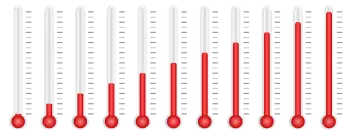# What Are the Main Temperature Scales?

Temperature scales (or thermometric scales) are the scales used to determine the temperature of a body by measuring it in degrees. A temperature scale is a method of expressing the physical quantity temperature in a number.Temperature is a scalar quantity that measures the amount of thermal energy that a body has.

There are two types of temperature scales:

• Empirical scales. These scales are based on the measurement of physical parameters,  usually a simple linear, functional relationship.

• Thermodynamic scales. These scales are absolute and are based on the fundamental laws of thermodynamics or statistical mechanics.

There are several temperature scales of which the most important are:

## 1. Kelvin Scale

The Kelvin scale is a centigrade scale to measure thermodynamic temperature whose unit of measurement is the kelvin. The kelvin is the base unit of temperature in the International System of Units (SI) and has the unit symbol K.

By convention, the zero of the Kelvin scale (absolute zero) corresponds to a temperature of -273.16 ° C. This value is the lowest possible temperature at which, in theory, there is no type of movement between the particles that make up matter.

The melting temperature of ice (corresponding to 273.16 K) and the boiling temperature of water (corresponding to 373.16 K) are taken as references on the Kelvin scale.

The interval between these two temperatures (373.16 K and 273.16 K) is divided into 100 equal parts, each of which is called Kelvin (and not degrees Kelvin). The Kelvin scale is the temperature scale used in the International System.

The absolute temperature scale is based on the (p, V, T) relationships for an ideal gas as given by equation (1.7)

PV=nRT

T is the absolute temperature, P, V, and n are the pressure, volume, and the number of moles as described, and R is the gas constant.

## 2. Celsius Scale

The Celsius scale was proposed in 1742 by the Swedish astronomer Anders Celsius.

On the Celsius scale, the freezing point of water corresponds to 0 ° C, while the boiling temperature of water corresponds to 100 degrees Celsius in a standard atmosphere. Both temperatures are determined at atmospheric pressure.

The interval between these freezing and boiling points (100 ° C and 0 ° C) is divided into 100 equal parts, called degrees Celsius or degrees centigrade. The Celsius scale is the temperature scale used in Europe.

One degree Celsius is equivalent to one kelvin.

The Celsius temperature scale has replaced the old Fahrenheit in Europe and most of the world. In thermodynamics, the absolute temperature scale is mainly used.

Since 1743 the Celsius scale has been based on 0 degrees Celsius for the freezing point of water and 100 degrees Celsius for the boiling point of water. Before 1743, the values were reversed.

## 3. Fahrenheit Scale

The Fahrenheit scale is a temperature scale proposed by the German physicist and engineer Daniel Gabriel Fahrenheit in 1724.

On the Fahrenheit temperature scale, the melting temperature of ice (which corresponds to 32 ° F) and the boiling point of water (which corresponds to 212 ° F) are taken as references.

The interval between these two temperatures (212 ° F and 32 ° F) is divided into 180 parts, known as degrees Fahrenheit. The Fahrenheit scale is the thermometric scale used in the United States and Anglo-Saxon countries.

## 4. Rankine Scale

The Rankin temperature scale is a scale for measuring temperatures proposed in 1859 by the Scottish engineer and physicist William John Macquorn Rankine.

° R. usually denotes the degree on this scale, Although it coincides with the notation for the degree of the Réaumur scale.

Like the Kelvin temperature scale, the Rankin scale is absolute; zero-degree is the absolute zero temperature. Unlike Kelvin, which took the Celsius size of the interval (100 ° between the melting point of ice and the boiling point of water at normal atmospheric pressure), Rankine took the magnitude of the degree Fahrenheit (180 ° between the melting point of ice and boiling point of water at normal atmospheric pressure).

## Disused Temperature Scales

Additionally to these four temperature scales, there are other disused temperature scales:

### Réaumur Scale

Degree Réaumur (ºRé), in disuse. It is due to Rene-Antoine Ferchault de Réaumur (1680-1757). The relationship with the Celsius scale is:

T (ºRé) = (4/5) * t (° C) or t (° C) = (5/4) * T (ºRé)

T (ºRé) = (4/5) * [T (K) - 273, 16] or T (K) = (5/4) * T (ºRé) + 273, 16

where T (ºRé) is the temperature expressed in degrees Réaumur.

### Rømer Scale

The unit of measurement on this scale (ºRø) is equivalent to 40/21 of a Kelvin (or one degree Celsius). The symbol for degree Rømer is ºRø.

T (ºRø) = (21/40) * t (° C) + 7.5 or t (° C) = (40/21) * [T (ºRø) - 7.5]

T (ºRø) = (21/40) * [T (K) - 273, 16] + 7, 5 or T (K) = (40/21) * [T (ºRø) - 7, 5] + 273, 16

where T (ºRø) is the temperature expressed in degrees Rømer.

### Delisle Scale

It was created by the French astronomer Joseph-Nicolas Delisle. Its units are degrees Delisle (or De Lisle), the symbol ºDe represents them, and each one is worth -2/3 of a degree Celsius or Kelvin. The zero of the scale is at the water's boiling temperature, and it increases as the other scales descend until it reaches absolute zero at 559.725ºDe.

### Newton Scale

T (ºN) = (33/100) * t (° C) or t (° C) = (100/33) * T (ºN)

T (ºN) = (33/100) * T (K) - 273, 16 or T (K) = (100/33) * T (ºN) + 273, 16

where T (ºN) is the temperature expressed in degrees Newton.

### Leiden Scale

Leiden degree (ºL) was used to calibrate indirectly low temperatures. Currently in disuse.

Author:

Published: June 8, 2021
Last review: June 8, 2021